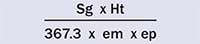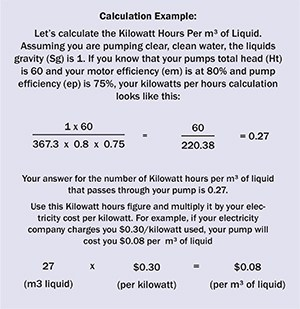As power costs rise, it is more vital than ever to know how much power your pump is consuming. This handy guide shows you how.

To calculate the cost of running your pump, we need two figures:

1.   The number of kilowatt hours per m³ of liquid, and

2.   Cost per kilowatt

Kilowatt Hours Per m³ of Liquid

You can calculate this by using the following formula:Where

Sg = specific gravity of liquid

Ht = pump total head in meters

367.3  = constant

em = motor efficiency at duty point as a decimal

ep = pump efficiency at duty point as a decimal

Cost per Kilowatt

Check you electricity bill for what your electricity company charges you per kilowatt of electricity used.Related articles